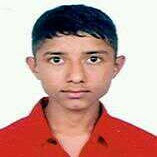# Fifth Semester Syllabus - PROBABILITY AND STATISTICS SH 602 B.Agri III/I

PROBABILITY AND STATISTICS
SH 602
Lecture     :   3                                                                                          Year   :   III
Tutorial     :   1                                                                                           Part   :   I
Practical   :   0
Course Objective:
To provide the students with practical knowledge of the principles and concept of probability and statistics and their applications in engineering field.

1.       Descriptive Statistics and Basic Probability                                             [6 hours]
1.1.  Introductions in statistics and its importance in engineering
1.2.  Describing data with graphs (bar, pie, line diagram, box plot)
1.3.  Describing data with numerical measure (measuring center, measuring variability)
1.4.    Basic probability additive law, multiplic active law, Baye’s theorem
2.       Discrete Probability Distributions                                                             [6 hours]
2.1.  Binomial probability distributions
2.2.  Negative binomial distribution
2.3.  Poison distribution
2.4.  Hyper geometric distributions
3.       Continuous Probability Distributions                                                       [6 hours]
3.1.  Continuous random variable and probability densities
3.2.  Normal distribution
3.3.  Gama distribution
3.4.  Chi-square distribution
4.       Sampling Distribution                                                                                  [5 hours]
4.1.  Population and sample
4.2.  Central limit theorems
4.3.  Sampling distribution of sampling proportion
4.4.  Sampling distributing of sampling proportion
5.       Correlation and regression                                                                        [6 hours]
5.1.  Least square methods
5.2.  An analysis of variance of linear regression model
5.3.  Inferences concerning least square method
5.4.  Multiple correlation and regression
6.       Inference concerning mean                                                                      [6 hours]
6.1.  Point estimation and interval estimation
6.2.  Test of hypothesis
6.3.  Hypothesis test concerning one mean
6.4.  Hypothesis test concerning two mean
6.5.  One way ANOVA
7.       Inference concerning proportion                                                            [6 hours]
7.1.  Estimation of proportions
7.2.  Hypotheses concerning one proportion
7.3.  Hypotheses concerning two proportions
7.4.  Chi-square test of independence
8.       Application of computer on statistical data computing                        [4 hours]
8.1.    Application of computer in computing statistical problem e.g. scientific
8.2.  Calculator, EXCEL, SPSS, Matlab, etc.

References:
1.     Richard A. Johnson, “Probability and statistics for engineers 7th edition, Miller and Freund’s publication
2.     Jay L devorce, probability and statistics for engineering and the sciences, brooks/ Cole publishing company, Monterey, California, 1982.
3.     Richard. Levin, David s Rubin, statistics for management. Prentice hall publication
4.     Mendenhall beaver, introduction probability and statistics 12th edition, Thomson brooks/Cole

Evaluation scheme:
The questions will cover the entire chapter of the syllabus. The evaluation scheme will be as indicated in the table below:
 Chapter Teaching hour Marks distribution* 1 6 12 2 6 10 3 6 10 4 6 10 5 6 10 6 6 10 7 6 10 8 4 8 Total 45 80
* There may be mirror deviation in marks distribution.

Ø  The questions setting should be in the multiplication of either 8 or 10.
###### Share To: